Ex 11.1

Chapter 11 Class 10 Constructions [Deleted]
Serial order wise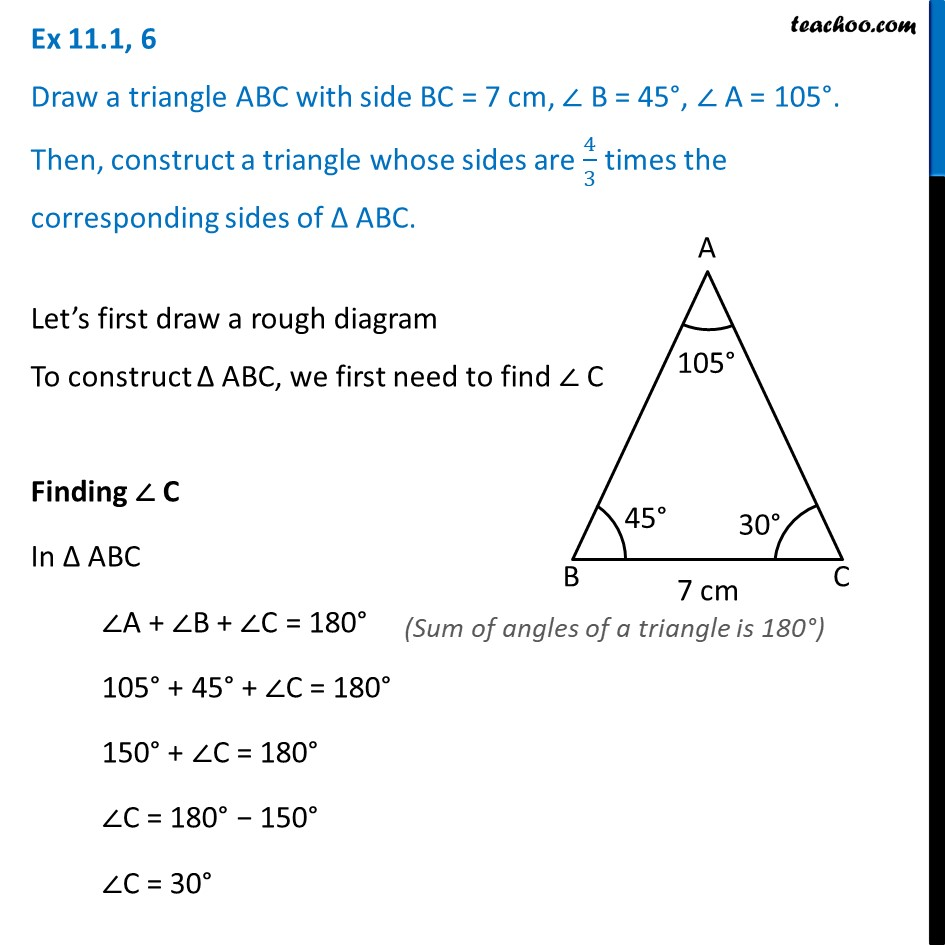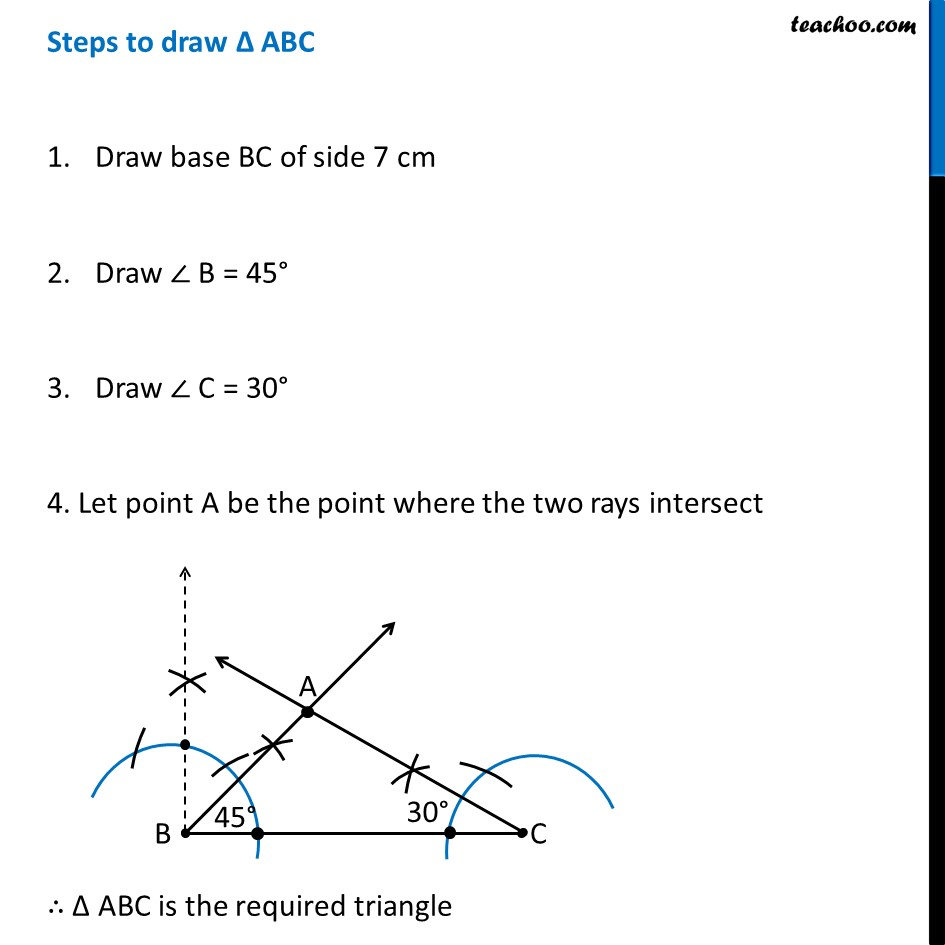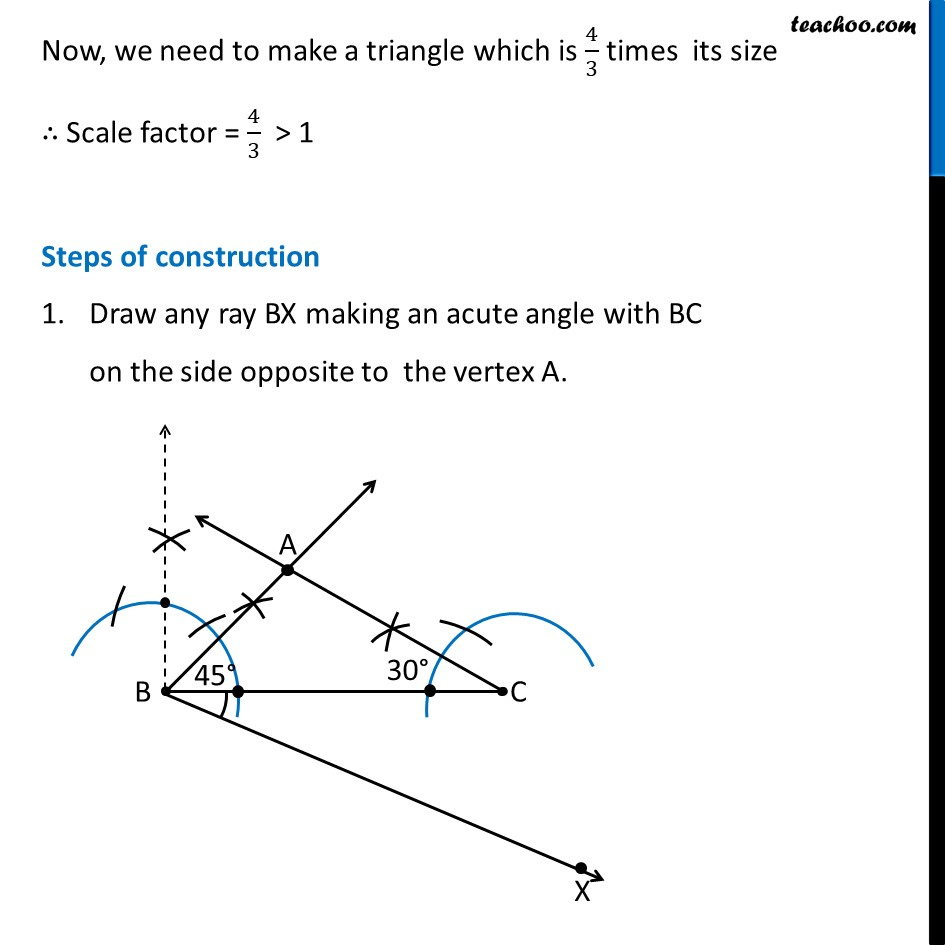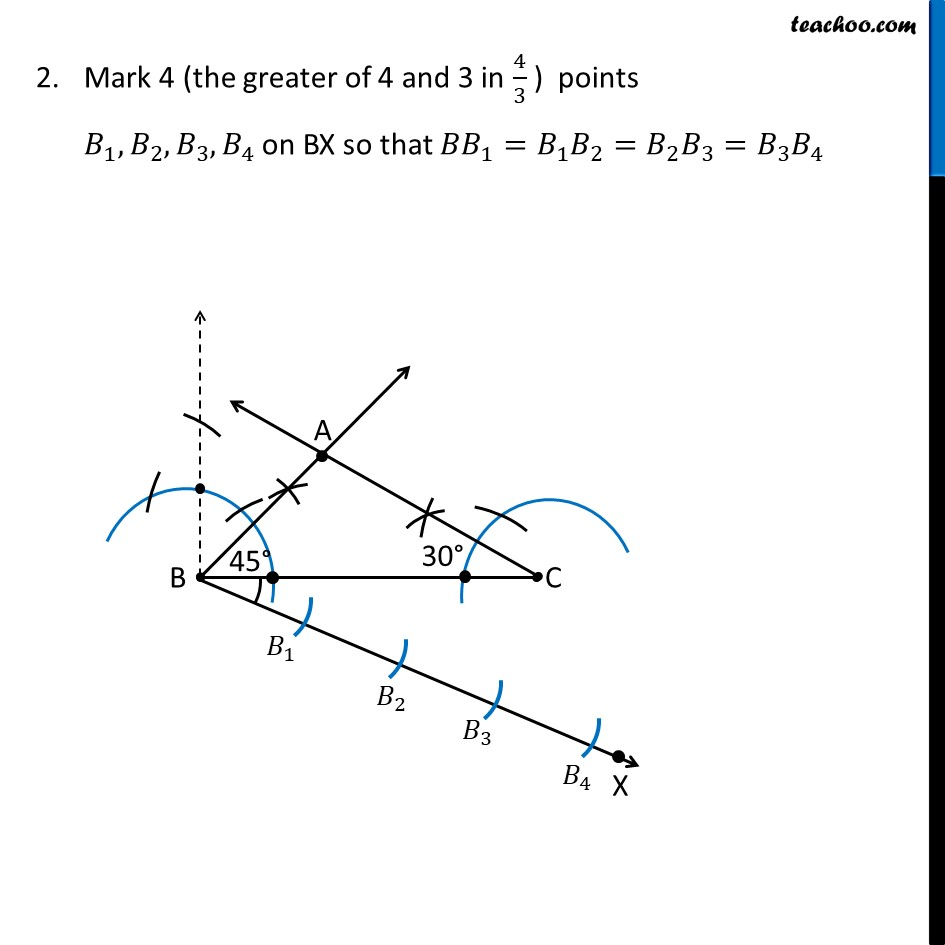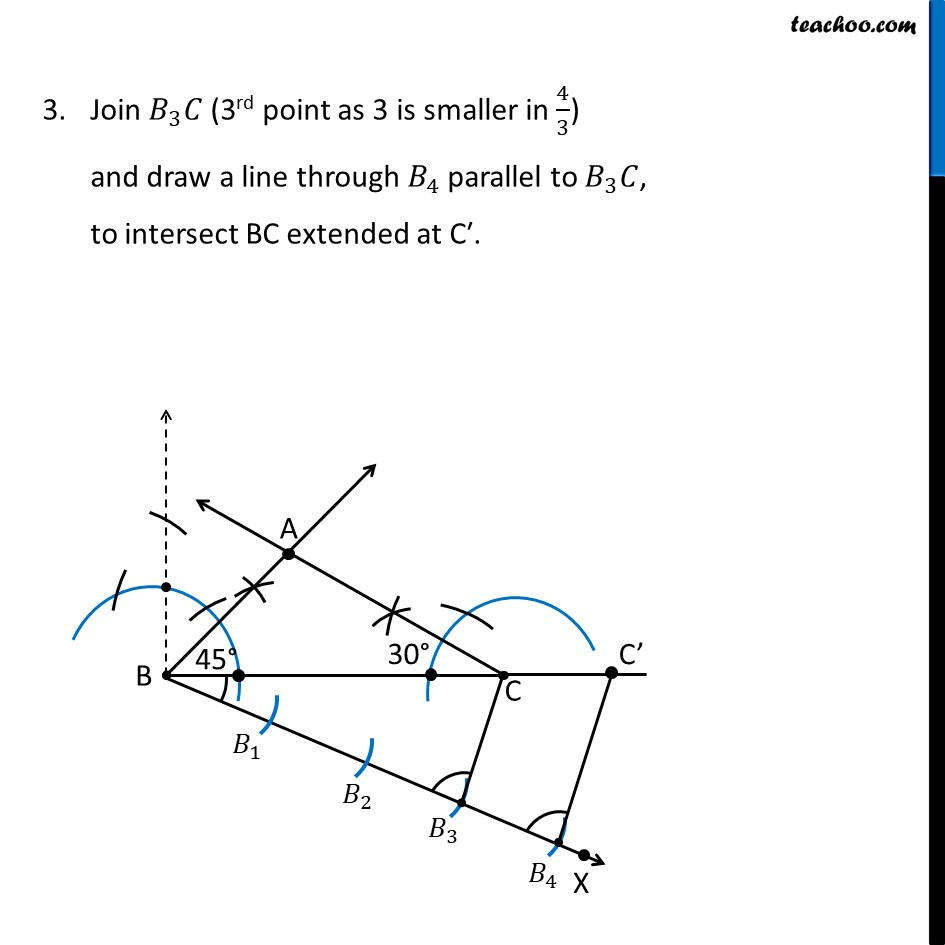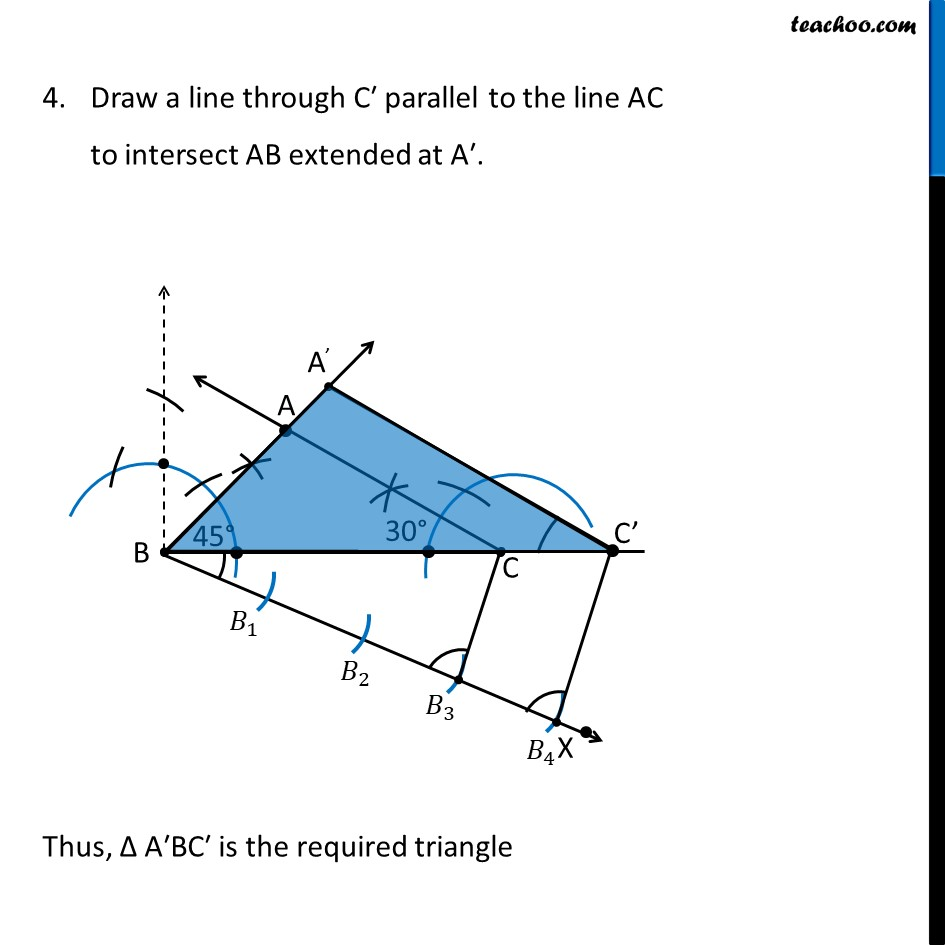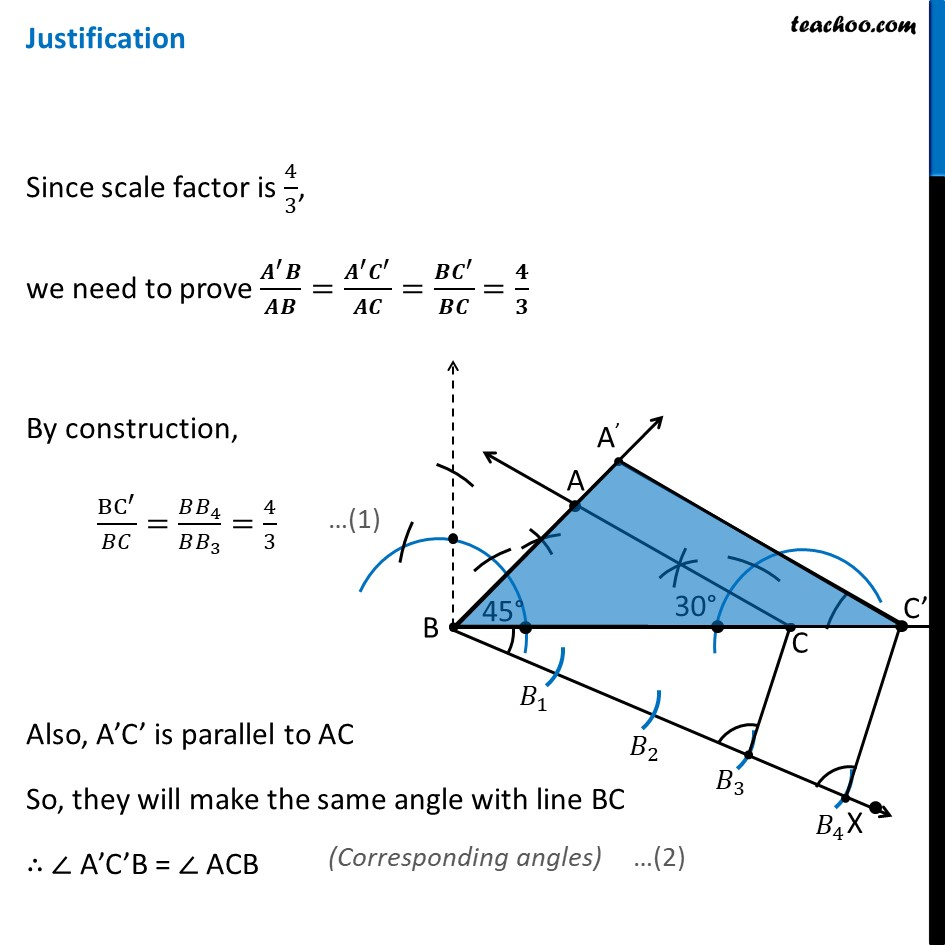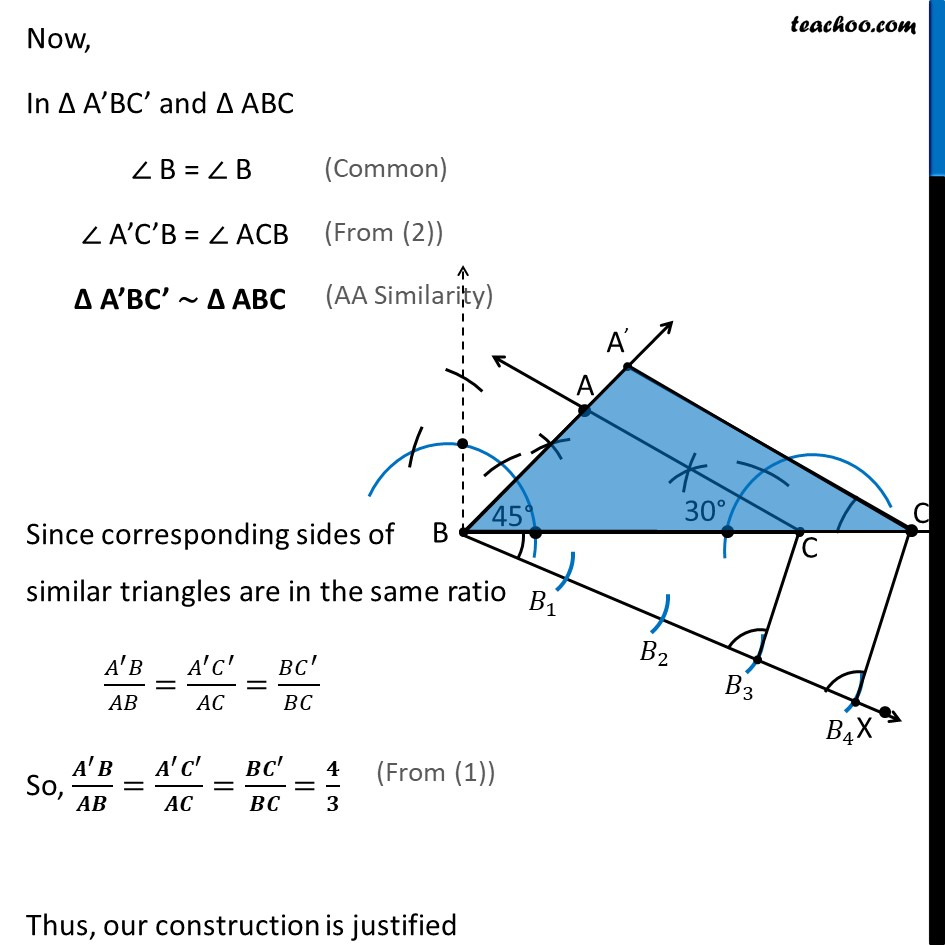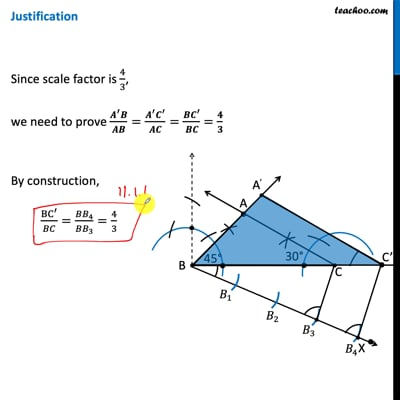This video is only available for Teachoo black users

Get live Maths 1-on-1 Classs - Class 6 to 12

### Transcript

Ex 11.1, 6 Draw a triangle ABC with side BC = 7 cm, ∠ B = 45°, ∠ A = 105°. Then, construct a triangle whose sides are 4/3 times the corresponding sides of Δ ABC. Let’s first draw a rough diagram To construct Δ ABC, we first need to find ∠ C Finding ∠ C In Δ ABC ∠A + ∠B + ∠C = 180° (Sum of angles of a triangle is 180°) 105° + 45° + ∠C = 180° 150° + ∠C = 180° ∠C = 180° − 150° ∠C = 30° Steps to draw Δ ABC Draw base BC of side 7 cm Draw ∠ B = 45° Draw ∠ C = 30° 4. Let point A be the point where the two rays intersect ∴ Δ ABC is the required triangle Now, we need to make a triangle which is 4/3 times its size ∴ Scale factor = 4/3 > 1 Steps of construction Draw any ray BX making an acute angle with BC on the side opposite to the vertex A. Mark 4 (the greater of 4 and 3 in 4/3 ) points 𝐵_1, 𝐵_2, 𝐵_3,𝐵_4 on BX so that 〖𝐵𝐵〗_1=𝐵_1 𝐵_2=𝐵_2 𝐵_3=𝐵_3 𝐵_4 Join 𝐵_3 𝐶 (3rd point as 3 is smaller in 4/3) and draw a line through 𝐵_4 parallel to 𝐵_3 𝐶, to intersect BC extended at C′. Draw a line through C′ parallel to the line AC to intersect AB extended at A′. Thus, Δ A′BC′ is the required triangle Justification Since scale factor is 4/3, we need to prove (𝑨^′ 𝑩)/𝑨𝑩=(𝑨^′ 𝑪^′)/𝑨𝑪=(𝑩𝑪^′)/𝑩𝑪 =𝟒/𝟑 By construction, BC^′/𝐵𝐶=(𝐵𝐵_4)/(𝐵𝐵_3 )=4/3 Also, A’C’ is parallel to AC So, they will make the same angle with line BC ∴ ∠ A’C’B = ∠ ACB Now, In Δ A’BC’ and Δ ABC ∠ B = ∠ B ∠ A’C’B = ∠ ACB Δ A’BC’ ∼ Δ ABC Since corresponding sides of similar triangles are in the same ratio (𝐴^′ 𝐵)/𝐴𝐵=(𝐴^′ 𝐶^′)/𝐴𝐶=(𝐵𝐶^′)/𝐵𝐶 So, (𝑨^′ 𝑩)/𝑨𝑩=(𝑨^′ 𝑪^′)/𝑨𝑪=(𝑩𝑪^′)/𝑩𝑪 =𝟒/𝟑 Thus, our construction is justified